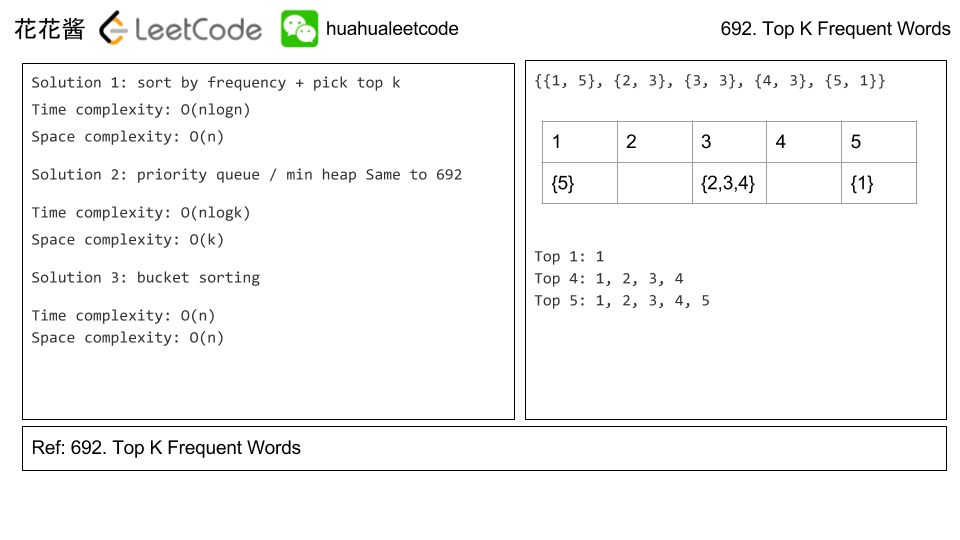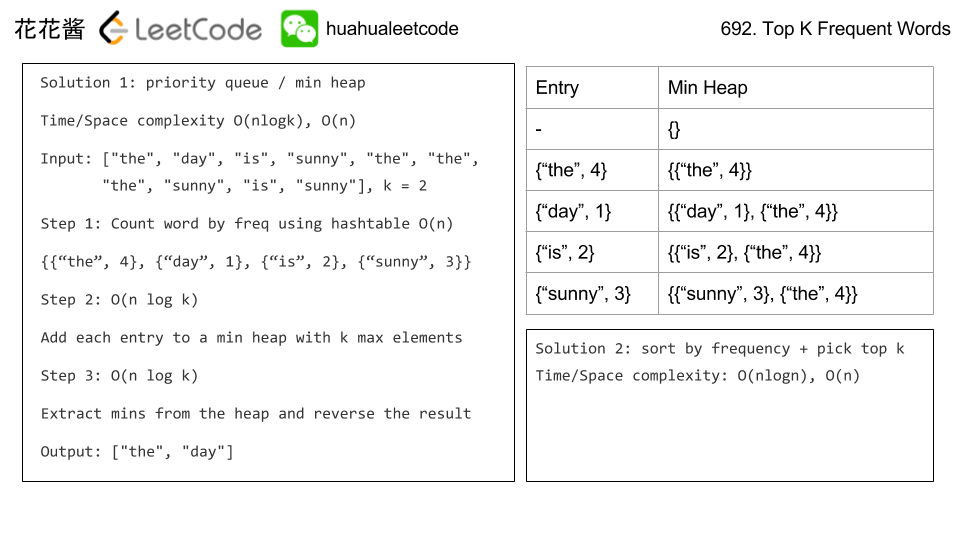# Posts tagged as “frequency”

With respect to a given puzzle string, a word is valid if both the following conditions are satisfied:

• word contains the first letter of puzzle.
• For each letter in word, that letter is in puzzle.
For example, if the puzzle is “abcdefg”, then valid words are “faced”, “cabbage”, and “baggage”; while invalid words are “beefed” (doesn’t include “a”) and “based” (includes “s” which isn’t in the puzzle).

Return an array answer, where answer[i] is the number of words in the given word list words that are valid with respect to the puzzle puzzles[i].

Example :

Input:
words = ["aaaa","asas","able","ability","actt","actor","access"],
puzzles = ["aboveyz","abrodyz","abslute","absoryz","actresz","gaswxyz"]
Output: [1,1,3,2,4,0]
Explanation:
1 valid word for "aboveyz" : "aaaa"
1 valid word for "abrodyz" : "aaaa"
3 valid words for "abslute" : "aaaa", "asas", "able"
2 valid words for "absoryz" : "aaaa", "asas"
4 valid words for "actresz" : "aaaa", "asas", "actt", "access"
There're no valid words for "gaswxyz" cause none of the words in the list contains letter 'g'.


Constraints:

• 1 <= words.length <= 10^5
• 4 <= words[i].length <= 50
• 1 <= puzzles.length <= 10^4
• puzzles[i].length == 7
• words[i][j]puzzles[i][j] are English lowercase letters.
• Each puzzles[i] doesn’t contain repeated characters.

## Solution: Subsets

Preprocessing:
Compress each word to a bit map, and compute the frequency of each bit map.
Since there are at most |words| bitmaps while its value ranging from 0 to 2^26, thus it’s better to use a hashtable instead of an array.

Query:
Use the same way to compress a puzzle into a bit map.
Try all subsets (at most 128) of the puzzle (the bit of the first character is be must), and check how many words match each subset.

words = [“aaaa”,”asas”,”able”,”ability”,”actt”,”actor”,”access”],
puzzle = “abslute”
bitmap(“aaaa”) = {0}
bitmap(“asas”) = {0, 18}
bitmap(“able”) = {0,1,4,11}
bitmap(“actt”) = {0, 2, 19}
bitmap(“actor”) = {0, 2, 14, 17, 19}
bitmap(“access”) = {0, 2, 4, 18}

bitmap(“abslute”) = {0, 1, 4, 11, 18, 19, 20}

Time complexity: O(sum(len(w_i)) + |puzzles|)
Space complexity: O(|words|)

## C++

Given a string s, we make queries on substrings of s.

For each query queries[i] = [left, right, k], we may rearrange the substring s[left], ..., s[right], and then choose up to k of them to replace with any lowercase English letter.

If the substring is possible to be a palindrome string after the operations above, the result of the query is true. Otherwise, the result is false.

Return an array answer[], where answer[i] is the result of the i-th query queries[i].

Note that: Each letter is counted individually for replacement so if for example s[left..right] = "aaa", and k = 2, we can only replace two of the letters.  (Also, note that the initial string s is never modified by any query.)

Example :

Input: s = "abcda", queries = [[3,3,0],[1,2,0],[0,3,1],[0,3,2],[0,4,1]]
Output: [true,false,false,true,true]
Explanation:
queries : substring = "d", is palidrome.
queries : substring = "bc", is not palidrome.
queries : substring = "abcd", is not palidrome after replacing only 1 character.
queries : substring = "abcd", could be changed to "abba" which is palidrome. Also this can be changed to "baab" first rearrange it "bacd" then replace "cd" with "ab".
queries : substring = "abcda", could be changed to "abcba" which is palidrome.


Constraints:

• 1 <= s.length, queries.length <= 10^5
• 0 <= queries[i] <= queries[i] < s.length
• 0 <= queries[i] <= s.length
• s only contains lowercase English letters.

## Solution: Prefix frequency

Compute the prefix frequency of each characters, then we can efficiently compute the frequency of each characters in the substring in O(1) time. Count the number odd frequency characters o, we can convert it to a palindrome if o / 2 <= k.

Time complexity:
preprocessing: O(n)
Query: O(1)
Space complexity: O(n)

## C++

Problem:

Find the minimum length word from a given dictionary words, which has all the letters from the string licensePlate. Such a word is said to complete the given string licensePlate

Here, for letters we ignore case. For example, "P" on the licensePlate still matches "p" on the word.

It is guaranteed an answer exists. If there are multiple answers, return the one that occurs first in the array.

The license plate might have the same letter occurring multiple times. For example, given a licensePlate of "PP", the word "pair" does not complete the licensePlate, but the word "supper" does.

Example 1:

Example 2:

Note:

1. licensePlate will be a string with length in range [1, 7].
2. licensePlate will contain digits, spaces, or letters (uppercase or lowercase).
3. words will have a length in the range [10, 1000].
4. Every words[i] will consist of lowercase letters, and have length in range [1, 15].

Idea:

Hashtable

Solution:

C++

Time complexity: 时间复杂度 O(N*26), N is number of words.

Space complexity: 空间复杂度 O(26) = O(1)

Problem:

Given a non-empty array of integers, return the k most frequent elements.

For example,
Given [1,1,1,2,2,3] and k = 2, return [1,2].

Note:
You may assume k is always valid, 1 ≤ k ≤ number of unique elements.
Your algorithm’s time complexity must be better than O(n log n), where n is the array’s size.

Idea:# Solution 2: Priority queue / max heap

Time complexity: O(n) + O(nlogk)

Space complexity: O(n)

# Solution 3: Bucket Sort

Time complexity: O(n)

Space complexity: O(n)

# Related Problems

Problem:

Given a non-empty list of words, return the k most frequent elements.

Your answer should be sorted by frequency from highest to lowest. If two words have the same frequency, then the word with the lower alphabetical order comes first.

Example 1:

Example 2:

Note:

1. You may assume k is always valid, 1 ≤ k ≤ number of unique elements.
2. Input words contain only lowercase letters.

1. Try to solve it in O(n log k) time and O(n) extra space.

Idea:

Priority queue / min heapSolution

C++ / priority_queue O(n log k) / O(n)

Related Problems

Mission News Theme by Compete Themes.